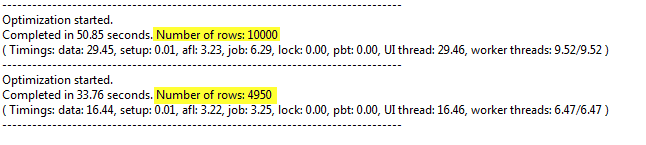#amibroker

### Using Exclude statement to skip unwanted optimization steps

Sometimes when we optimize our system, we may want to use only a subset of all parameter permutations for our analysis and ignore the others that do not meet our requirements.

For example – if we test a simple trend-following strategy, where we enter long position when short MA crosses above long MA using code such as:

`shortPeriods = Optimize("Short MA", 10, 1, 100, 1);longPeriods = Optimize("long MA", 50, 1, 100, 1);Buy = Cross( MA( Close, shortPeriods), MA( Close, longPeriods) );Sell = Cross( MA( Close, longPeriods), MA( Close, shortPeriods) )`

Then, shortPeriods parameter value should remain smaller than longPeriods, otherwise the trading rules would work against the main principle of the tested strategy.

There is an easy way to ignore the unwanted sets of parameters by using Exclude statement in our code. If the variable is true – the backtester will not calculate any statistics for that particular run:

`shortPeriods = Optimize("Short MA", 10, 1, 100, 1);longPeriods = Optimize("long MA", 50, 1, 100, 1);Buy = Cross( MA( Close, shortPeriods), MA( Close, longPeriods) );Sell = Cross( MA( Close, longPeriods), MA( Close, shortPeriods) );Exclude = shortPeriods >= longPeriods`

The information from Info tab of Analysis window shows the difference between first execution (all 10000 backtest runs) and second one using Exclude statement. Note reduced number of steps and reduced optimization time.Comments are closed.